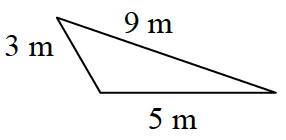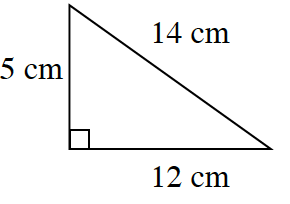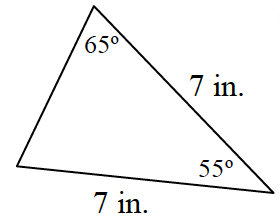### Home > CCG > Chapter 3 > Lesson 3.1.1 > Problem3-9

3-9.

Examine each diagram below. Identify the error in each diagram.1.Compare the length of the longest side with the sum of the two shorter sides.

1.Test the side lengths with the Pythagorean Theorem.

1.Since the sum of the interior angles in a triangle is $180^\circ$, fill in the measure of the third angle. Considering the given side lengths, does this make sense?